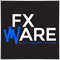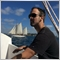# Moving average of an indicator25

Hi,

I'm new in programming MQL4, I'm trying to calculate the mving average of an indicator in an EA.

Could someone give me a code example of :

- The moving average of x period of the volume of a symbol ?

- The moving average of x period of the slow stochastic(5,10,3) of a symbol ?25

No one to help me ?.249

I would imagine it would have something to do with re-writing a custom MA indicator that looks at average values of the indicator you want. Should really just be a case of replacing the price values in the current MA indicator with iStochastic values then you can just use iCustom() to call the custom indicator. I think.

here's the simple moving average code from the MA indicator that comes with MT4, should just be a case of replacing the Close[pos] with iStochastic(...,[pos]) values i think

void sma()
{
double sum=0;
int i,pos=Bars-ExtCountedBars-1;
//---- initial accumulation
if(pos<MA_Period) pos=MA_Period;
for(i=1;i<MA_Period;i++,pos--)
sum+=Close[pos];
//---- main calculation loop
while(pos>=0)
{
sum+=Close[pos];
ExtMapBuffer[pos]=sum/MA_Period;
sum-=Close[pos+MA_Period-1];
pos--;
}
//---- zero initial bars
if(ExtCountedBars<1)
for(i=1;i<MA_Period;i++) ExtMapBuffer[Bars-i]=0;25

mrwobbles:

I would imagine it would have something to do with re-writing a custom MA indicator that looks at average values of the indicator you want. Should really just be a case of replacing the price values in the current MA indicator with iStochastic values then you can just use iCustom() to call the custom indicator. I think.

here's the simple moving average code from the MA indicator that comes with MT4, should just be a case of replacing the Close[pos] with iStochastic(...,[pos]) values i think

void sma()
{
double sum=0;
int i,pos=Bars-ExtCountedBars-1;
//---- initial accumulation
if(pos<MA_Period) pos=MA_Period;
for(i=1;i<MA_Period;i++,pos--)
sum+=Close[pos];
//---- main calculation loop
while(pos>=0)
{
sum+=Close[pos];
ExtMapBuffer[pos]=sum/MA_Period;
sum-=Close[pos+MA_Period-1];
pos--;
}
//---- zero initial bars
if(ExtCountedBars<1)
for(i=1;i<MA_Period;i++) ExtMapBuffer[Bars-i]=0;

Thanks for your help, mrwobbles, but it didn't help me yet.

Imagine simply I would like to calculate the moving average of x periods on volumes, have you got the code for this ?249

I've done a simple MA of the volume, if you look at the Moving Averages indicator that comes with MT4 you can see what I've done. It's fairly simple. The problem with the Stochastic MA is that stochastics return positive and negative values so the sums arent accurate.
Files:249

here's one that has an exponential MA in it as well. just change MA method, 0=Simple MA, 1=Exponential MA. Oh and you have to set the colour to red, default is black for some reason...
Files:6

Use iMAOnArray() function. For the data array, use an array you previously populated with the indicator's data.

PS:

Note that

ArraySetAsSeries(array, true);

is necessary before calling iMAOnArray function.611

As said ahmetozer10  you have to use iMAOnArray()

e.g.

```int period = 7;
int history = 100;
double array[];
ArrayResize(array,history);

for (int i=0; i<history; i++) array[i]=(double)iVolume(NULL,0,i);

double result = iMAOnArray(array,0,period,0,MODE_SMA, shift);  // shift = studied bar```6

Note that

ArraySetAsSeries(array, true);

is necessary before calling iMAOnArray function.471

ahmetozer10:
Use iMAOnArray() function. For the data array, use an array you previously populated with the indicator's data.

PS:

Note that

ArraySetAsSeries(array, true);

is necessary before calling iMAOnArray function.

You have just answered 10 years old question. I know, theoretically there is possibility they guy still is looking for an answer but the chances are slim ;)86

paul selvan:

As said ahmetozer10  you have to use iMAOnArray()

e.g.

How do I do this on MQL5 the iMAOnArray() is not available.

Im trying to find the 20MA of the On Balance Volume. Am I missing something.

I am new to this type of thing.

```//+------------------------------------------------------------------+
//|   On Balance Volume                                              |
//+------------------------------------------------------------------+
// create an Array for several prices
double OnBalanceVolumeArray[];
double OnBalance_MA_Array[];

// sort the price array from the current candle downwards
ArraySetAsSeries(OnBalanceVolumeArray,true);
// sort the price array1 from the current candle downwards
ArraySetAsSeries(OnBalance_MA_Array,true);

// define the properties of the OBV
int OBVDefinition = iOBV (_Symbol,_Period,VOLUME_TICK);
int OBV_MA20 = iMA(   ? )

// Defined OBV, one line,current candle,20 candles, store result
CopyBuffer(OBVDefinition,0,0,20,OnBalanceVolumeArray);
// Defined OBV, one line,current candle,20 candles, store result
CopyBuffer(OBV_MA20,0,0,20,OnBalance_MA_Array);```